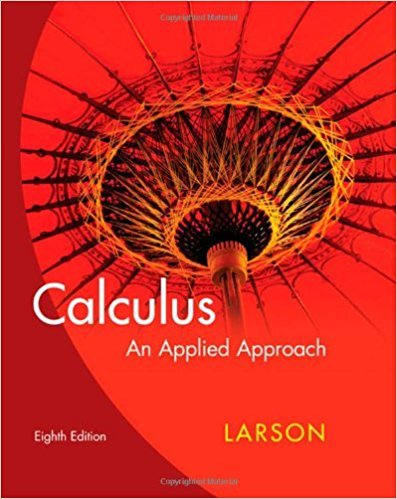×
×

# Solutions for Chapter 8: Trigonometric Functions## Full solutions for Calculus: An Applied Approach | 8th Edition

ISBN: 9780618958252Solutions for Chapter 8: Trigonometric Functions

Solutions for Chapter 8
4 5 0 319 Reviews
11
2
##### ISBN: 9780618958252

This expansive textbook survival guide covers the following chapters and their solutions. Since 118 problems in chapter 8: Trigonometric Functions have been answered, more than 23861 students have viewed full step-by-step solutions from this chapter. Calculus: An Applied Approach was written by and is associated to the ISBN: 9780618958252. This textbook survival guide was created for the textbook: Calculus: An Applied Approach , edition: 8. Chapter 8: Trigonometric Functions includes 118 full step-by-step solutions.

Key Calculus Terms and definitions covered in this textbook
• Cofunction identity

An identity that relates the sine, secant, or tangent to the cosine, cosecant, or cotangent, respectively

• Convergence of a sequence

A sequence {an} converges to a if limn: q an = a

• Correlation coefficient

A measure of the strength of the linear relationship between two variables, pp. 146, 162.

• Distance (on a number line)

The distance between real numbers a and b, or |a - b|

• Graph of a relation

The set of all points in the coordinate plane corresponding to the ordered pairs of the relation.

• Graph of an equation in x and y

The set of all points in the coordinate plane corresponding to the pairs x, y that are solutions of the equation.

• Limaçon

A graph of a polar equation r = a b sin u or r = a b cos u with a > 0 b > 0

• Logarithmic re-expression of data

Transformation of a data set involving the natural logarithm: exponential regression, natural logarithmic regression, power regression

• Multiplication property of inequality

If u < v and c > 0, then uc < vc. If u < and c < 0, then uc > vc

• Multiplicative inverse of a matrix

See Inverse of a matrix

• nth root of a complex number z

A complex number v such that vn = z

• Parameter interval

See Parametric equations.

• Parametric equations

Equations of the form x = ƒ(t) and y = g(t) for all t in an interval I. The variable t is the parameter and I is the parameter interval.

• Placebo

In an experimental study, an inactive treatment that is equivalent to the active treatment in every respect except for the factor about which an inference is to be made. Subjects in a blind experiment do not know if they have been given the active treatment or the placebo.

• Present value of an annuity T

he net amount of your money put into an annuity.

The measure of a central angle whose intercepted arc has a length equal to the circle’s radius.

• Rigid transformation

A transformation that leaves the basic shape of a graph unchanged.

• Rose curve

A graph of a polar equation or r = a cos nu.

• Second-degree equation in two variables

Ax 2 + Bxy + Cy2 + Dx + Ey + F = 0, where A, B, and C are not all zero.

• Series

A finite or infinite sum of terms.

×